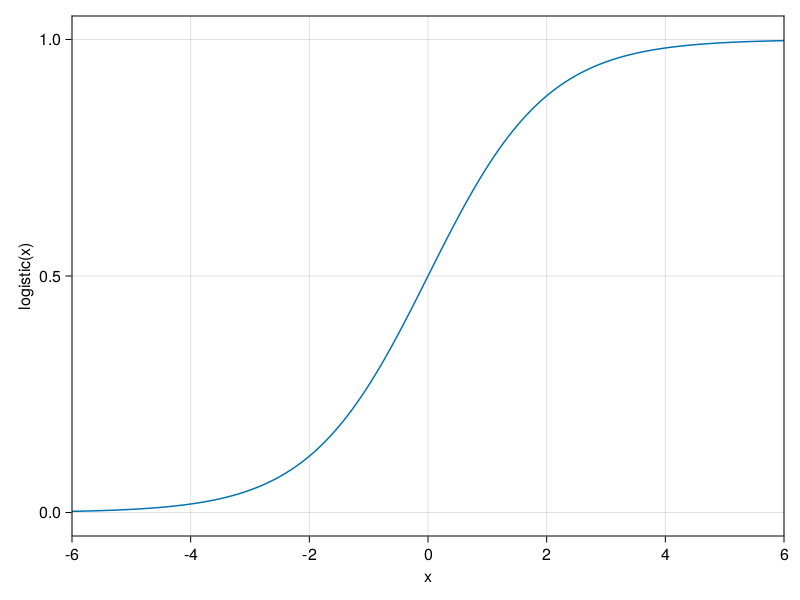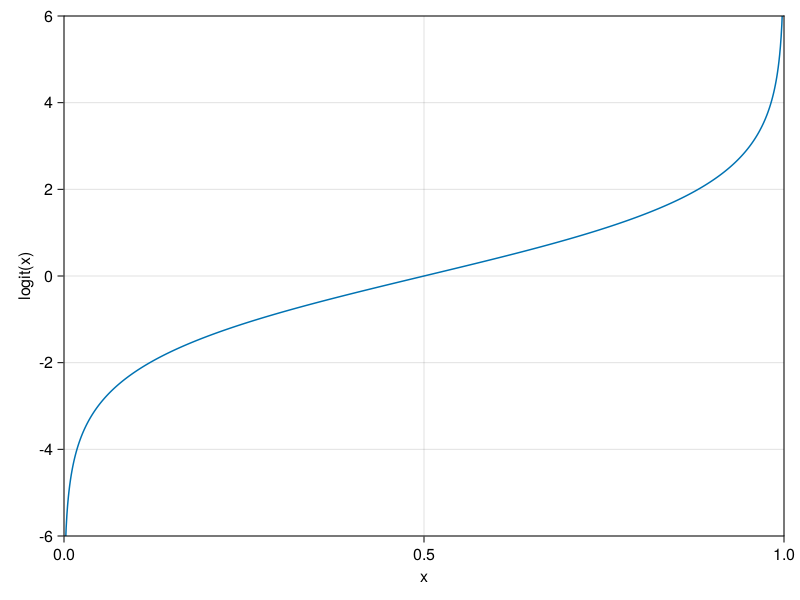HUIJZER.XYZ

# The logit and logistic functions

2020-11-04

Linear regression works on real numbers $\mathbb{R}$, that is, the input and output are in $\mathbb{R}$. For probabilities, this is problematic because the linear regression will happily give a probability of $-934$, where we know that probabilities should always lie between $0$ and $1$. This is only by definition, but it is an useful definition in practice. Informally, the logistic function converts values from real numbers to probabilities and the logit function does the reverse.

## Logistic

The logistic function converts values from $(-\infty, \infty)$ to $(0, 1)$:

$$\text{logistic}(x) = \frac{1}{1 + e^{-x}}.$$

We can easily define this function ourselves:

mylogistic(x) = 1 / (1 + exp(-x));

But, also load it from StatsFuns.jl:

using StatsFuns: logistic
mylogistic(1) == logistic(1)
true

Graphically, this looks as follows:If you care enough, you could decide to remember the plot by heart. Some people advise to remember the following numbers by heart.

\begin{aligned} \text{logistic}(-3) &\approx 0.05, \\ \text{logistic}(-1) &\approx \tfrac{1}{4}, \\ \text{logistic}(1) &\approx \tfrac{3}{4}, \: \text{and} \\ \text{logistic}(3) &\approx 0.95. \end{aligned}

since

r2(x) = round(x; digits=2);
logistic(-3) |> r2
0.05
logistic(-1) |> r2
0.27
logistic(1) |> r2
0.73
logistic(3) |> r2
0.95

## Logit

The inverse of the logistic function is the logit function:

$$\text{logit}(x) = \log\frac{x}{1 - x}$$

mylogit(x) = log(x / (1 - x));
using StatsFuns: logit
logit(1) == mylogit(1)
true

This function goes from $(0, 1)$ to $(-\infty, \infty)$:Built with Julia 1.9.3 and

CairoMakie 0.10.5
StatsFuns 1.3.0

To run this blog post locally, open this notebook with Pluto.jl.